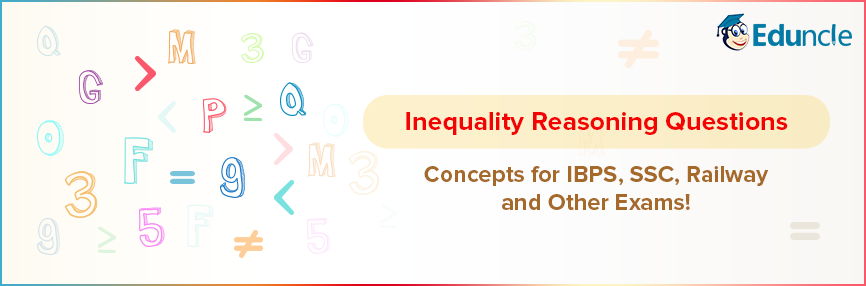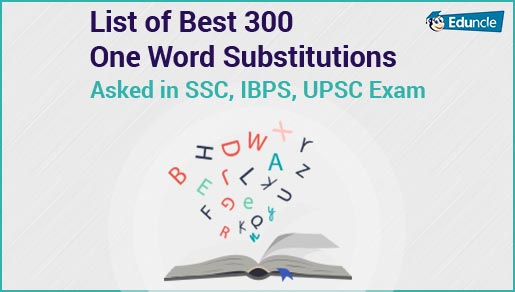Government Jobs FollowRavi @Eduncle posted an Article
May 27, 2019 • 3:08 pm

# Inequality Questions Concepts for IBPS, SSC, Railway and Other Exams!Inequality is one of the easiest and scoring topic of Reasoning Section.

Candidates can score well by spending less time on this topic in competitive exams like IBPS PO, IBPS Clerk, SBI PO, RRB, SSC CGL etc.

This topic is considered as the easiest one to score in the exam. But one must have practiced well to crack Inequality questions.

There are certain rules that should be followed in each Inequality Problem.

Solving Inequality questions will become easy when you understand the basic concepts and rules of Inequality.

Let’s discuss the general terms and rules of Inequality that helps an aspirant to attempt Inequality Problems.

Targeting Upcoming SSC Exams 2018-2019? Get Free Study Materials and Previous Year Question Papers. Download Now.

## What is Inequality Reasoning? A Proper Definition!

Relationship between more than 2 elements which is stated by some particular symbol is inequality.

Questions with a statement consisting of group of elements can be separated by inequality symbols like >, < and =.

Following are the five science or we can say five possibilities which are used to describe the relationship between certain objects.

 Signs Meaning > Greater than < Less than ≥ Greater than or Equal to ≤ Less than or Equal to = Equal to ≠ Inequal to

To understand the use of above-mentioned symbols in Inequality problems, below we have shared an example for you.

Let’s assume there are two variables X and Y. How many conditions can be made between X and Y by using the Inequality symbols.

X > Y means the value of X is greater than Y.

X < Y means the value of X is less than Y.

X ≥ Y means the value of X is greater than or equal to Y.

X ≤ Y means the value of X is less than or equal to Y.

X = Y means the value of X is equal to the value of Y.

X ≠ Y means X is either greater than or less than Y.

The complete Inequality problem is based on the above-mentioned symbols.

As you are now well aware of the basics of Inequality. Here, we are sharing the important Inequality equation rules that are used to solve questions of IBPS PO, IBPS Clerk, SSC and other exams.

Start Preparation of Bank Exams 2019 with Free Study Stuff - Download Now!

## Inequality Rules - Know How You Can Crack Inequality Questions!

The Inequality questions are always based on different number of elements and the numbers of symbols are also clubbed accordingly.

Check the relation and conclusion as per the given condition.

### #Rule – 1

The combination between two Inequalities for common terms

 Condition Relation or Conclusion A > B, B > C Relation – A > B > C Conclusion A > C or C < A A < B, B < C Relation – A < B < C Conclusion – A < C or C > A A ≥ B, B ≥ C Relation – A ≥ B ≥ C Conclusion – A ≥ C or C ≤ A

### #Rule – 2

The combination between the two elements is not possible if they are not common terms.

 Condition Relation or Conclusion A > B, C > B Relation - Cannot define specific relation between A and C. Conclusion – Both A and C are greater than B. B > A, D < B Relation – Cannot define specific relation between A and D. Conclusion – Both A and D are less than B. A ≥ B, B ≤ C Relation – Cannot define specific relation between A and C. Conclusion – Both A and C are greater than or equal to B.

### #Rule – 3

The third important rule is, if the common term is greater than or “greater than or equal to” one and less than or ‘less than or equal to’ the other, the combination between two inequality can be established.

Condition 1

A ≥ B, C < B.

Here in the given elements, we can easily establish the combination.

Here B is the common term which is related to the other two terms.

So, the possible relations are –

A ≥ B > C or C < B ≤ A

Conclusion

A > C or C < A.

Condition 2

A ≥ B, B < C

Here C is the common term, but we can not make combination between the other elements of the given relation.

Condition 3

A ≥ B, C ≥ A

Here A is the common term than the other two elements.  A is greater than and equal to B and less than and equal to C.  So, the establishment of elements is possible with these three elements.

The possible relations between these three elements are –

C ≥ A ≥ B or B ≤ A ≤ C

Conclusion

C > A or B < C

Condition 4

A ≥ B, A ≥ C

From this condition, we cannot establish the combination in between given elements as the common term A is greater than both the remaining elements.

### #Rule – 4

The common elements in which no relationship is established, cannot be combined

This condition occurs in Complementary Pair (Either & or) cases.

Let’s understand it with a perfect example –

The statement is –

A ≥ B, B ≤ C

Conclusion

1. A ≥ C

2. A < C

The relationship can not be established in between A and C as per the given statement.

You can only say that either A is greater than or equal to C or you can say A is less than C.

So, you can only choose either condition in both the conclusion.

Vedic Math Tricks

Start Preparation of Bank Exams 2018-19 with Free Study Stuff & Video Lectures - Download Now!

## Coded Inequalities Questions – Know the Concept Here!

Nowadays there is a new pattern of inequality questions asked in exams which has become a trend.

We are talking about the Coded Inequalities questions which are mostly asked in almost every Government Jobs exam.

In such type of questions, the usual mathematical symbols are used in statements, unlike the usual ones.

So, how you can solve such type of Inequality questions? To know the concepts, just scroll down the blog.

The basic concept to solve coded inequality questions is to know the meaning of given signs in a question.

You will be required to substitute the real signs in the given expression to solve the question.

The BODMAS rules will play an important role in solving coded inequalities.

The symbols used in coded inequality questions can be like %, ∆, #, &, ¢, @, #, $, ©. To understand the concepts of coded Inequality here we bring a perfect example for you. Check this below. Instructions The symbols$, @, #, ^, and * are used in the following question are illustrated below.

P $Q means ‘P is not smaller than Q’. P * Q means ‘P is not greater than Q’. P # Q means ‘P is neither greater than nor equal to Q’. P ^ Q means ‘P is neither greater than nor smaller than Q’. P @ Q means ‘P is neither smaller than nor equal to Q’. In each of the below-given statements, assume that the statements are true. Find which of the conclusion is/are definitely true. Question Statement H @ T, T # F, F ^ E, E * V Conclusions I. V$ F

II.         E @ T

III.       H @ V

IV.       T # V

(A) Only I, II and III are true

(B) Only I, II and IV are true

(C) Only I, III and IV are true

(D) Only II, III and IV are true

(E) All I, II, III and IV are true

Solution

Based on the direction as given in instructions, we can substitute the symbols like –

H > T, T < F, F = E, E ≤ V

The relation that we can make in all these elements is –

H > T < F = E ≤ V

As per the coded inequalities rules and the available options, we can see that option I, II and IV are correct.

So, here the conclusion is (B) Only I, II and IV are true.

Prepare for Upcoming SSC Exams! Download Here to get free study materials PDFs and Sample Papers for all exams.

Here we are ending up with this blog. We tried to provide all the required information, tips, and tricks that can help you to crack the questions based on Inequality.

Hope you will use these tricks to solve the practice sets of Inequality questions to boost your overall score in competitive exams.

Queries are meant to be asked. So, use the comments’ box to get the solutions of each of your problem by experts.

You Might Also Like